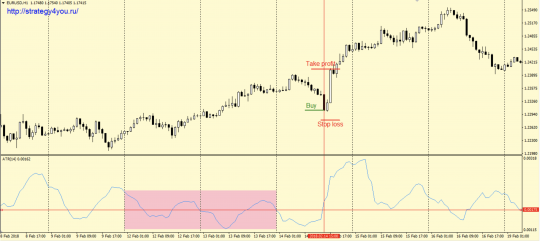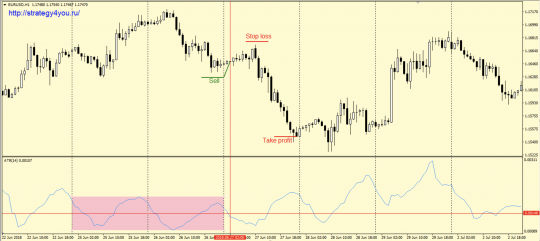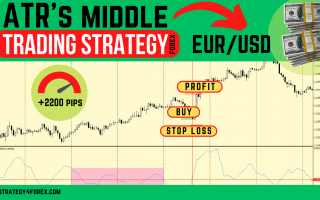# +2200 points — Forex strategy “ATR’s middle”

Forex strategy «ATR’s middle», as the name implies, is based on the average level of the ATR indicator. In fact, signals can be received both with and against the trend, which does not prevent you from making a profit. Another interesting feature of this strategy is the fact that there are much more sell transactions, however, this aspect does not affect the effectiveness of the trading system.

Statistics:

Over the past year, from July 2017 to July 2018, the strategy showed a result in the region of +2200 points. The maximum drawdown did not exceed 150 points (all data in points were calculated using 4-digit quotes).

• Currency pair — eur/usd.
• Time interval — H1.

Indicators:

• ATR indicator with a period of 14.

## Conditions for purchases according to the rules of the «ATR’s middle» forex strategy:1) At the close of the next day, you should highlight the range of movement of the ATR indicator for the previous two days (from maximum to minimum).

2) Then the middle of this range is calculated and a horizontal line is drawn along it.

3) If the first crossing of this line occurs from the bottom up, then at the close of the candle, on which the ATR indicator is fixed above the center line, a buy deal is concluded.

4) Stop loss is 25 points.

5) After passing 25 points in the positive zone, the transaction is transferred to breakeven.

6) Take profit is 100 pips.

## Conditions for the sale of the vehicle «ATR’s middle»:1) At the close of the day, you should highlight the range of movement of the ATR indicator for the previous two days (from high to low).

2) Then the middle of this range is calculated (the middle of the pink rectangle in the chart above) and a horizontal line (red) is drawn along it.

3) If the first crossing of this line occurs from top to bottom, then at the close of the candle, on which the ATR indicator is fixed below the center line, a sell deal is concluded.

4) Set a safety stop loss at a distance of 25 points.

5) After passing 25 points in the «+» zone, the transaction is transferred to the breakeven point.

6) Take profit order is equal to 100 points.

### Video version of the «ATR’s middle» forex strategy:

• Recommended to watch with English subtitles!